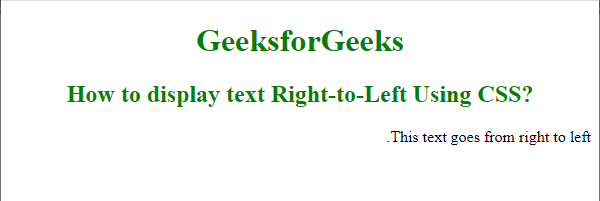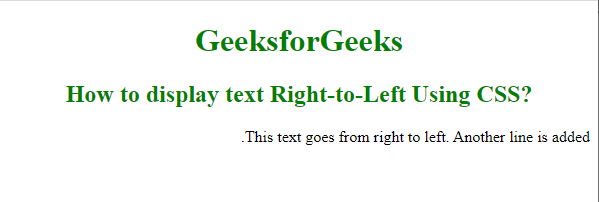GeeksforGeeks App
Open AppBrowser
Continue

# How to display text Right-to-Left Using CSS ?

In this article, we will learn how to display text Right-to-Left using CSS. This is done with the help of the CSS Direction property. By default, the text alignment is set to left to right direction in HTML Document.  To display the text from right to left, we simply set the direction property to the value of “rtl”.

Syntax:

```element_selector {
direction: rtl;
} ```

Example 1: Here is the basic implementation of the CSS direction property.

## HTML

 ```<``html``>``<``head``>``    ``<``style``>``        ``h1,``        ``h2 {``            ``color: green;``            ``text-align: center;``        ``}` `        ``.rtl {``            ``direction: rtl;``        ``}``    ````` `<``body``>``    ``<``h1``>``        ``GeeksforGeeks``    ``` `    ``<``h2``>``        ``How to display text``        ``Right-to-Left Using CSS?``    ``` `    ``<``p` `class``=``"rtl"``>``        ``This text goes from right to left.``    ```````

Output:Right to left

Example 2: In the above code, if we add some more text content in the “p” element, we get the following output. Notice the effect in both results.

## HTML

 ```<``html``>``<``head``>``    ``<``style``>``        ``h1,``        ``h2 {``            ``color: green;``            ``text-align: center;``        ``}` `        ``.rtl {``            ``direction: rtl;``        ``}``    ````` `<``body``>``    ``<``h1``>``        ``GeeksforGeeks``    ``` `    ``<``h2``>``        ``How to display text Right-to-Left Using CSS?``    ``` `    ``<``p` `class``=``"rtl"``>``        ``This text goes from right to left.``        ``Another line is added.``    ```````

Output:Right to left

My Personal Notes arrow_drop_up
Related Tutorials